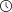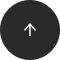- 4 minutes read

# Why Aren’t SQL Window Functions Allowed in GROUP BY?

It’s true – the SQL order of operations means window functions aren’t allowed in GROUP BY. But you can use them anyway with this special trick.

SQL window functions are one of the language’s most powerful features. However, the syntax of window functions is not easy to master. It has lots of details that can cause beginners to stumble. One of the most common traps is trying to use window functions in `GROUP BY`.

## Error: Window Functions Are Not Allowed in GROUP BY

Imagine you have a table, `midterm`, that stores the results of a mid-term test taken by students. The columns in the table are the `name` of the student and the number of `points` the student got on the test. You’d like to split the students into four groups of equal size based on their test result: the top 25% are in one group, the next 25% in the second group, the third 25% in the third group, and the bottom 25% in the last group. Then you’d like to see the range of points and the number of students in each group.

Here’s a query you could write:

```SELECT
ntile(4) OVER (ORDER BY points),
min(points),
max(points),
count(*)
FROM midterm
GROUP BY ntile(4) OVER (ORDER BY points);
```

The `NTILE()` function divides students into groups and assigns each student the number (1-4) of their group. We then want to group students based on the number of their group and compute the minimum, maximum, and count for each group. However, when you run this query, you’ll get an error:

```ERROR:  window functions are not allowed in GROUP BY
LINE 7: GROUP BY ntile(4) OVER (ORDER BY points);
```

## Why Window Functions Aren’t Allowed in GROUP BY

The reason why window functions are not allowed in `GROUP BY` is the order of operations in SQL. The clauses of a SQL query are processed in a different order than they are written in the query. The full order of operation in SQL is:

• `FROM`, `JOIN`
• `WHERE`
• `GROUP BY`
• Aggregate functions
• `HAVING`
• Window functions
• `SELECT`
• `DISTINCT`
• `UNION`/`INTERSECT`/`EXCEPT`
• `ORDER BY`
• `OFFSET`
• `LIMIT`/`FETCH`/`TOP`

A SQL query first figures out the tables to query from, then it applies the `WHERE` filters, and then it performs the `GROUP BY` operation. Then it proceeds to compute aggregate functions, `HAVING` filters, and finally window functions. So, at the time the `GROUP BY` is evaluated, the window functions are not yet computed!

In practice, you can only directly refer to SQL window functions in the `SELECT` and `ORDER BY` clauses.

The order of operations in SQL is one of the most important things to remember when you write a query with window functions. If you don’t use window functions often, it’s easy to forget this, which is why we put a reminder of the order of operations in our SQL Window Functions Cheat Sheet. Make sure to bookmark it if you use window functions every once in a while!

So, SQL doesn’t let you put window functions in a `GROUP BY`. But there happens to be a way around this ...

## How to Use Window Functions in GROUP BY

Can we fix the query so that it returns the result we want? Yes. The solution is to use a subquery to compute the window function you want to use in the main query. Here’s our modified example:

```SELECT
quartile,
min(points),
max(points),
count(*)
FROM
(SELECT
ntile(4) OVER (ORDER BY points) AS quartile,
points
FROM midterm) groups
GROUP BY quartile;
```

In the subquery, we use the `NTILE()` function to assign students into groups. In the main query, we compute the statistics: the minimum, maximum, and the number of students.

Another possibility is to use a common table expression (CTE), like so:

```WITH groups AS (
SELECT
ntile(4) OVER (ORDER BY points) AS quartile,
points
FROM midterm
)
SELECT
quartile,
min(points),
max(points),
count(*)
FROM groups
GROUP BY quartile;
```

The query is similar to the previous version with the subquery. However, a CTE lets us define the auxiliary query before the main query; this makes the code more readable.

## Master Window Functions with LearnSQL.com

If you want to learn window functions, we recommend our interactive Window Functions course. You’ll learn how to use window functions and how to avoid common rookie mistakes. If you’re not sure if window functions are for you, read why you should learn them or this article about our Window Functions course.